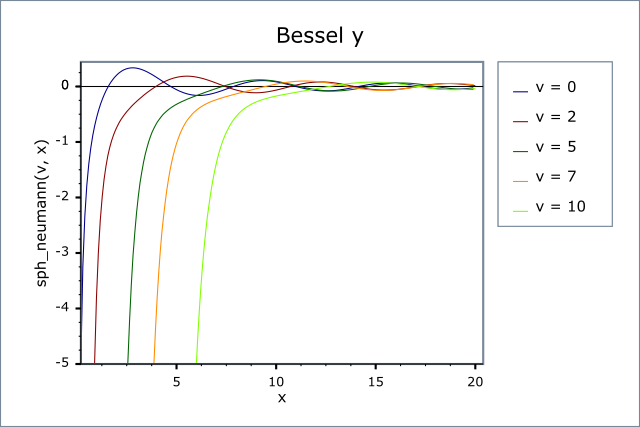#Boost C++ Libraries

...one of the most highly regarded and expertly designed C++ library projects in the world.

This is the documentation for an old version of boost. Click here for the latest Boost documentation.

#### Spherical Bessel Functions of the First and Second Kinds

##### Synopsis
```template <class T1, class T2>
calculated-result-type sph_bessel(unsigned v, T2 x);

template <class T1, class T2, class Policy>
calculated-result-type sph_bessel(unsigned v, T2 x, const Policy&);

template <class T1, class T2>
calculated-result-type sph_neumann(unsigned v, T2 x);

template <class T1, class T2, class Policy>
calculated-result-type sph_neumann(unsigned v, T2 x, const Policy&);
```
##### Description

The functions sph_bessel and sph_neumann return the result of the Spherical Bessel functions of the first and second kinds respectively:

sph_bessel(v, x) = jv(x)

sph_neumann(v, x) = yv(x) = nv(x)

where:The return type of these functions is computed using the result type calculation rules for the single argument type T.

The final Policy argument is optional and can be used to control the behaviour of the function: how it handles errors, what level of precision to use etc. Refer to the policy documentation for more details.

The functions return the result of domain_error whenever the result is undefined or complex: this occurs when `x < 0`.

The jv   function is cyclic like Jv   but differs in its behaviour at the origin:Likewise yv   is also cyclic for large x, but tends to -∞   for small x:##### Testing

There are two sets of test values: spot values calculated using functions.wolfram.com, and a much larger set of tests computed using a simplified version of this implementation (with all the special case handling removed).

##### Accuracy

Other than for some special cases, these functions are computed in terms of cyl_bessel_j and cyl_neumann: refer to these functions for accuracy data.

##### Implementation

Other than error handling and a couple of special cases these functions are implemented directly in terms of their definitions:The special cases occur for:

j0  = sinc_pi(x) = sin(x) / x

and for small x < 1, we can use the series:which neatly avoids the problem of calculating 0/0 that can occur with the main definition as x → 0.

 Copyright © 2006-2010 John Maddock, Paul A. Bristow, Hubert Holin, Xiaogang Zhang, Bruno Lalande, Johan Råde, Gautam Sewani and Thijs van den Berg Distributed under the Boost Software License, Version 1.0. (See accompanying file LICENSE_1_0.txt or copy at http://www.boost.org/LICENSE_1_0.txt)